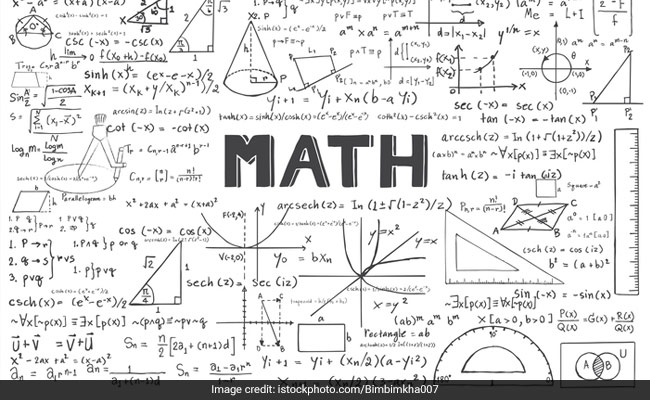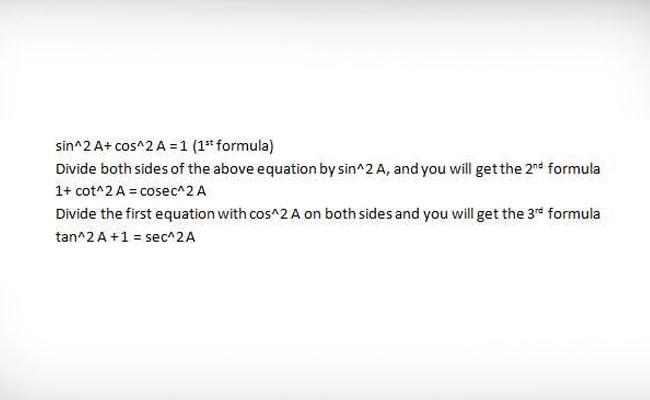Wednesday, 25 Dec 2019

# CBSE Board Exam 2019: Tips For Class 10th Geometry, TrigonometryBoard Exam for CBSE 10th class for mathematics exam is on March 7

Board Exam 2019: CBSE board exams have begun and with it have the last leg of preparation. The mathematics exam for class 10th students is scheduled on March 7. The last few days leading up to the CBSE Board exam demand a different preparation strategy form students. During this time, students should not try to study the entire syllabus and instead practice only the key points. Keeping this in mind we have curated some important last-minute tips for CBSE class 10th mathematics board exam focusing specifically on Trigonometry and Geometry.

Geometry and Trigonometry are topics which are easy to solve if the student has all the concepts clear in their mind. The key is to break down a problem to the most basic component and then solve it.

CBSE Board 2019: Revision, Examination Tips For Maths Exam

These tips are shared by Ashish Malik, an ex-student of the board, who completed an MS degree in Statistics from Purdue University, USA and says that these were his go to points when preparing for Mathematics exam in class 10.

1. When solving a problem, tray and convert everything to ‘sin and cos’. Solving trigonometric equations becomes easier.

2. Remember the three Square Law Formulas:Square Law Formulas in Trigonometry

3. While proving, try working the RHS to get some idea and solve backwards.

4. In questions where you have to prove LHS=RHS, remember that you can make any change in the LHS only and you cannot modify RHS.

5. Make sure that all the given information is translated into a diagram. Try making it as accurate as possible. Knowing the quadrants or points makes it easier to find the final answer.

6. Instead of using a name such as ABC, write ABC as 1 and so on. It will save you a lot of time. Sometimes it creates confusion when you have multiple angles in the said question like ABC, ACB etc. Always remember to mark the points, i.e. 1, 2 in the diagram.

7. Important properties of some quadrilaterals:

Parallelogram – if opposite sides are equal, diagonals are unequal

Rectangle – if opposite sides are equal and the two diagonals are also equal

Rhombus – if all sides are equal but diagonals are unequal

Square – if all sides are equal and diagonals are also equal